# Calculus 1 : How to find local maximum by graphing differential equations

## Example Questions

### Example Question #1 : How To Find Local Maximum By Graphing Differential Equations

Find the local maximum for the function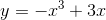.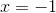Explanation:

To find the local max, you must find the first derivative, which is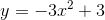.

Then. you need to set that equal to zero, so that you can find the critical points. The critical points are telling you where the slope is zero, and also clues you in to where the function is changing direction. When you set this derivative equal to zero and factor the function, you get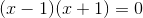, giving you two critical points atand.

Then, you set up a number line and test the regions in between those points. To the left of -1, pick a test value and plug it into the derivative. I chose -2 and got a negative value (you don't need the specific number, but rather, if it's negative or positive). In between -1 and 1, I chose 0 and got a possitive value. To the right of 1, I chose 2 and got a negative value. Then, I examine my number line to see where my function was going from positive to negative because that is what yields a maximum (think about a function going upwards and then changing direction downwards). That is happening at x=1.

### Example Question #1 : How To Find Local Maximum By Graphing Differential Equations

Find the local maximum of the function.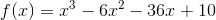None of these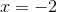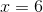Explanation:

When the derivative of a function is equal to zero, that means that the point is either a local maximum, local miniumum, or undefined. The derivative of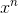is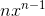. The derivative of the given function is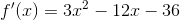We must now set it equal to zero and factor.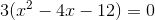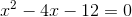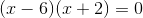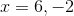Now we must plug in points to the left and right of the critical points to determine which is the local maximum.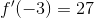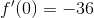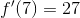This means the local maximum is atbecause the function is increasing at numbers less than -2 and decreasing at number between -2 and 6

### Example Question #3 : How To Find Local Maximum By Graphing Differential Equations

Find the local maximum of the function.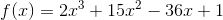None of these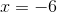Explanation:

The points where the derivative of a function are equal to 0 are called critical points. Critical points are either local maxs, local mins, or do not exist. The derivative ofis. The derivative of the function is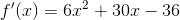Now we must set it equal to 0 and factor to solve.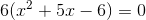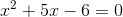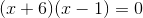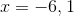We must now plug in points to the left and right of the critical points into the derivative function to figure out which is the local max.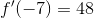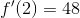This means that the function is increasing until it hits x=-6, then it decreases until x=1, then it begins increasing again.

This means that x=-6 is the local max.

### Example Question #4 : How To Find Local Maximum By Graphing Differential Equations

Find thecoordinate of the local maximum of the folowing function.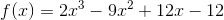None of theseExplanation:

At local maximums and minumims, the slope of the line tangent to the function is 0. To find the slope of the tangent line we must find the derivative of the function.

The derivative ofis. Thus the derivative of the function is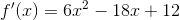To find maximums and minumums we set it equal to 0.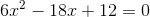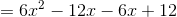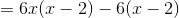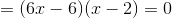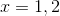So the critical points are at x=1 and x=2. To figure out the maximum we must plug each into the original function.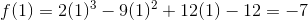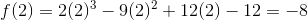So the local max is at x=1.